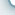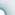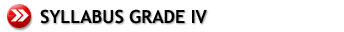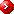Primary Mathematics U.S. Edition 4AWhole NumbersMultiplication and Division of Whole NumbersFractions Thousands, Hundreds, Tens and OnesNumbers to 100,000 Rounding off Numbers Factors Multiples Multiplication by a 1-digit Number Division by a 1-digit Number and by 10 Multiplication by a 2-digit Number Adding Fractions Subtracting Fractions Mixed Numbers Improper Fractions Fraction of a SetTables and Graphs Angle Perpendicular and Parallel Lines Presenting Data Measuring Angles Perpendicular Lines Parallel Lines Area and Perimeter Rectangles and Squares Composite Figures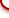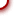Primary Mathematics U.S. Edition 4BDecimalsThe Four Operations of DecimalsMeasures Tenths Hundredths Thousandths Rounding off Addition and Subtraction Multiplication Division Multiplication DivisionSymmetry Solid Figures Volume Symmetric Figures Identifying Solid Figures Cubic Units Volume of a Cuboid Time Geometry Area and Perimeter Hours and Minutes Other Units of Time Angles Right Angles Area Perimeter Area of a RectanglecybeRShala Difference Lesson Illustration How It Works Compare Us Request Info Placement Test Tell a friend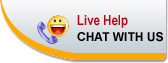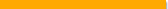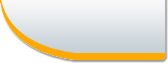Home | FAQ | Tell a Friend | Contact Us | Compare Us | Homeschool Resources | Sitemap cybershala.com Inc. All Rights reserved | Terms of service | Privacy policy